# C program to find the largest of two numbers

In this article, you will learn and get code about how to find and print the largest number between any given two numbers by the user (at run-time) with the following approaches:

## Using if-else logic, determine the greater of two numbers

To find the largest or greatest one between any given two numbers in C programming, you have to ask the user to enter any two numbers to check and find out which one is larger, as shown in the program given below:

```#include<stdio.h>
#include<conio.h>
int main()
{
int a, b, big;
printf("Enter any two number: ");
scanf("%d%d", &a, &b);
if(a>b)
big=a;
else
big=b;
printf("\nBiggest of the two number is: %d", big);
getch();
return 0;
}```

As the above program was written in the Code::Blocks IDE, therefore, after a successful build and run, it will produce the following result: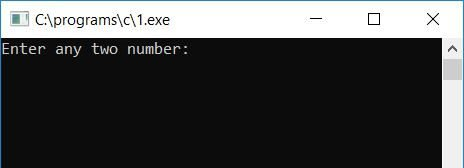Now you have to supply any two numbers, say 10 and 20, and then press ENTER to see the output as given in the second snapshot here. As we all know, 20 is greater than 10, therefore, you will see 20 as the output:If you want to test with the first number as 20 and the second number as 10, you can do so. Here is the output: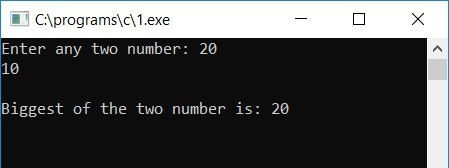#### Program Explained

• Take any two numbers and put them in two variables, say a and b.
• Check whether a is greater than b or not using the if statement.
• If it is, then the value of a will be initialized to big.
• Otherwise, the value of b will be initialized to big.
• Whatever the biggest value is, big holds it.
• Finally, print the value of the big variable as output.

## Find the largest of two numbers using the ternary operator

Here is another program that does the same job as the previous one. This program uses the ternary operator to find the greater one.

```#include<stdio.h>
#include<conio.h>
int main()
{
int num1, num2, large;
printf("Enter any two numbers: ");
scanf("%d%d", &num1, &num2);
large = (num1>num2) ? num1 : num2;
printf("\nLargest = %d", large);
getch();
return 0;
}```

Here is the first screenshot of the sample run: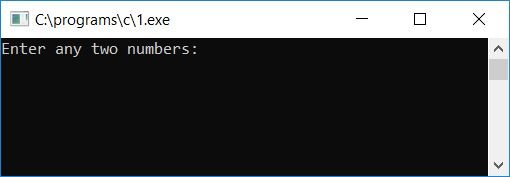Now supply any two numbers and press ENTER to see the largest number, as shown here in the second snapshot: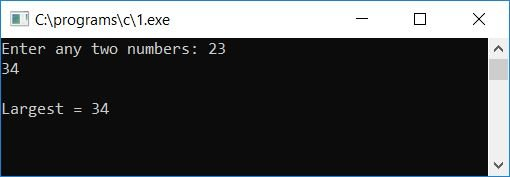The main logical code is:

`large = (num1>num2) ? num1 : num2;`

Here, if num1 is greater than num2, then num1 will be initialized to large, otherwise num2 will be initialized to large.

Or you can say that if num1 is greater than num2, then the whole expression after large = will become num1; therefore, the statement becomes:

`large = num1;`

Otherwise (if num1 is less than num2), the statement becomes:

`large = num2;`

## Find the largest of two numbers using a function

This is the final program in which I've written a function findLarge() that takes the user's number as an argument and returns the largest one.

```#include<stdio.h>
#include<conio.h>
int findLarge(int, int);
int main()
{
int num1, num2, larg;
printf("Enter any two numbers: ");
scanf("%d%d", &num1, &num2);
larg = findLarge(num1, num2);
printf("Largest = %d", larg);
getch();
return 0;
}
int findLarge(int a, int b)
{
if(a<b)
return b;
else
return a;
}```

Here is the final snapshot of the sample run: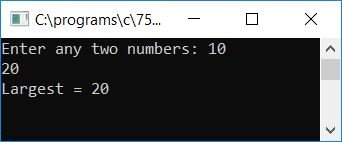Inside the function named findLarge(), if the value at b is greater than the value at a, then b will be returned and initialized to the large variable; otherwise, a will be returned and initialized to the large variable inside the main() function.

#### The same program in different languages

C Quiz

« Previous Program Next Program »